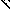# MINLPLib

### A Library of Mixed-Integer and Continuous Nonlinear Programming Instances

#### Instance: ex2_1_9

 Formatsⓘ ams gms lp mod nl osil pip Primal Bounds (infeas ≤ 1e-08)ⓘ -0.37500000 p1 ( gdx sol ) (infeas: 0) Other points (infeas > 1e-08)ⓘ Dual Boundsⓘ -0.37500000 (ANTIGONE)-0.37500000 (BARON)-0.37500000 (COUENNE)-0.37500060 (LINDO)-0.37500000 (SCIP) Referencesⓘ Floudas, C A, Pardalos, Panos M, Adjiman, C S, Esposito, W R, Gumus, Zeynep H, Harding, S T, Klepeis, John L, Meyer, Clifford A, and Schweiger, C A, Handbook of Test Problems in Local and Global Optimization, Kluwer Academic Publishers, 1999. Sourceⓘ Test Problem ex2.1.9 of Chapter 2 of Floudas e.a. handbook Added to libraryⓘ 31 Jul 2001 Problem typeⓘ QP #Variablesⓘ 10 #Binary Variablesⓘ 0 #Integer Variablesⓘ 0 #Nonlinear Variablesⓘ 10 #Nonlinear Binary Variablesⓘ 0 #Nonlinear Integer Variablesⓘ 0 Objective Senseⓘ min Objective typeⓘ quadratic Objective curvatureⓘ indefinite #Nonzeros in Objectiveⓘ 10 #Nonlinear Nonzeros in Objectiveⓘ 10 #Constraintsⓘ 1 #Linear Constraintsⓘ 1 #Quadratic Constraintsⓘ 0 #Polynomial Constraintsⓘ 0 #Signomial Constraintsⓘ 0 #General Nonlinear Constraintsⓘ 0 Operands in Gen. Nonlin. Functionsⓘ Constraints curvatureⓘ linear #Nonzeros in Jacobianⓘ 10 #Nonlinear Nonzeros in Jacobianⓘ 0 #Nonzeros in (Upper-Left) Hessian of Lagrangianⓘ 44 #Nonzeros in Diagonal of Hessian of Lagrangianⓘ 0 #Blocks in Hessian of Lagrangianⓘ 1 Minimal blocksize in Hessian of Lagrangianⓘ 10 Maximal blocksize in Hessian of Lagrangianⓘ 10 Average blocksize in Hessian of Lagrangianⓘ 10.0 #Semicontinuitiesⓘ 0 #Nonlinear Semicontinuitiesⓘ 0 #SOS type 1ⓘ 0 #SOS type 2ⓘ 0 Infeasibility of initial pointⓘ 0 Sparsity JacobianⓘSparsity Hessian of Lagrangianⓘ```\$offlisting
*
*  Equation counts
*      Total        E        G        L        N        X        C        B
*          2        2        0        0        0        0        0        0
*
*  Variable counts
*                   x        b        i      s1s      s2s       sc       si
*      Total     cont   binary  integer     sos1     sos2    scont     sint
*         11       11        0        0        0        0        0        0
*  FX      0
*
*  Nonzero counts
*      Total    const       NL      DLL
*         21       11       10        0
*
*  Solve m using NLP minimizing objvar;

Variables  x1,x2,x3,x4,x5,x6,x7,x8,x9,x10,objvar;

Positive Variables  x1,x2,x3,x4,x5,x6,x7,x8,x9,x10;

Equations  e1,e2;

e1.. -(x1*x2 + x2*x3 + x3*x4 + x4*x5 + x5*x6 + x6*x7 + x7*x8 + x8*x9 + x9*x10
+ x1*x3 + x2*x4 + x3*x5 + x4*x6 + x5*x7 + x6*x8 + x7*x9 + x8*x10 + x1*x9
+ x1*x10 + x2*x10 + x1*x5 + x4*x7) - objvar =E= 0;

e2..    x1 + x2 + x3 + x4 + x5 + x6 + x7 + x8 + x9 + x10 =E= 1;

* set non-default levels
x4.l = 0.25;
x5.l = 0.25;
x6.l = 0.25;
x7.l = 0.25;

Model m / all /;

m.limrow=0; m.limcol=0;
m.tolproj=0.0;

\$if NOT '%gams.u1%' == '' \$include '%gams.u1%'

\$if not set NLP \$set NLP NLP
Solve m using %NLP% minimizing objvar;

```

Last updated: 2021-03-11 Git hash: 66559cbc